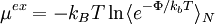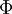# Widom test-particle methodThis article is a 'stub' page, it has no, or next to no, content. It is here at the moment to help form part of the structure of SklogWiki. If you add sufficient material to this article then please remove the {{Stub-general}} template from this page.$\mu^{ex} = -k_BT \ln \langle e^{-\Phi/k_bT}\rangle_N$
where$k_B$ is the Boltzmann constant, T is the temperature, N is the number of particles within the system and$\Phi$ is the interaction potential energy between the randomly placed test particle and the N particles that the system is comprised of.